# MCAT Physical : Endothermic and Exothermic Reactions

## Example Questions

← Previous 1

### Example Question #1 : Endothermic And Exothermic Reactions

Boiling point is the temperature a liquid needs to achieve in order to begin its transformation into a gaseous state. Campers and hikers who prepare food during their trips have to account for differences in atmospheric pressure as they ascend in elevation. During the ascent, the decrease in atmospheric pressure changes the temperature at which water boils.

Further complicating the matter is the observation that addition of a solute to a pure liquid also changes the boiling point. Raoult’s Law can be used to understand the changes in boiling point if a non-volatile solute is present, as expressed here.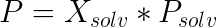In this law,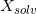is the mole fraction of the solvent,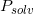is the vapor pressure of the pure solvent, andis the vapor pressure of the solution. When this vapor pressure is equal to the local atmospheric pressure, the solution boils.

A scientist is creating a solution to study vapor pressure. When she adds the solute, intermolecular bonds in the solute break to allow the solution to form. The formation of the solution is exothermic. Which of the following is true?

Breaking solute-solute bonds absorbs energy

Forming solute-solvent bonds absorbs energy

Forming solute-solute bonds absorbs energy

Breaking solvent-solvent bonds releases energy

Breaking solute-solute bonds releases energy

Breaking solute-solute bonds absorbs energy

Explanation:

Even though the dissolution process is exothermic, breaking bonds ALWAYS requires energy input. In the case of an exothermic dissolution, more energy is released when solute-solvent bonds are formed, than was absorbed when solute-solute bonds were broken. The initial step requires an input of activation energy, but the final product allows for a net energy release.

### Example Question #2 : Endothermic And Exothermic Reactions

When a solution is formed, which of the following is true?

Formation of a solution causes a decrease in entropy

Solution formation that is exothermic results in stronger intermolecular bonds, compared to the bonds in the pure substances

The bonds in the solute molecules are broken in an exothermic process

The bonds between the solvent molecules are broken in an exothermic process

None of these statements are true

Solution formation that is exothermic results in stronger intermolecular bonds, compared to the bonds in the pure substances

Explanation:

When dealing with the enthalpy of a solution, there are three specific steps, each having their own enthalpy.

1) The breaking of solute-solute

2) The breaking of solvent-solvent bonds

3) The formation of solute-solvent bonds

Remember that breaking bonds always requires an input of energy, and is thus considered endothermic. The formation of new bonds is an exothermic process. When an overall solution is exothermic, it means that the new intermolecular bonds are more stable. A system with less energy is considered more stable.

### Example Question #3 : Endothermic And Exothermic Reactions

The formation of nitrous oxide is a 2-step process.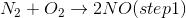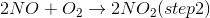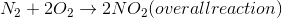The overall enthalpy of the reaction is +68kJ.

Given the above information, which of the following statements must be true?

The forward overall reaction has a higher activation energy than the reverse overall reaction.

Both of the steps are endothermic.

The overall reaction releases heat to the surroundings.

Both of the steps are exothermic.

The forward overall reaction has a higher activation energy than the reverse overall reaction.

Explanation:

When a reaction has a positive enthalpy change, it is said to be endothermic. This means that it requires an addition of heat into the system. Because the forward reaction requires more energy than the reverse reaction in order to take place, we see that the activation energy of the endothermic reaction is greater than the activation energy of the reverse reaction.

Essentially, the forward reaction requires a net input of energy, while the reverse reaction results in a net release of energy.

### Example Question #4 : Endothermic And Exothermic Reactions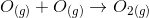Which of the following is true in the reaction above?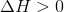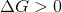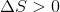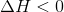Explanation:

The process of bond formation in chemistry is exothermic. By definition, exothermic reactions have a negative enthalpy change; therefore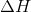must be less than zero in this bond-forming reaction.

Reducing moles of gas will decrease entropy, meaning that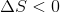. If both entropy and enthalpy are negative, as in this reaction, we cannot determine the value of Gibbs free energy without knowing the temperature.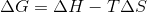### Example Question #5 : Endothermic And Exothermic Reactions

Based on the energy diagram, which chemical processes are exothermic?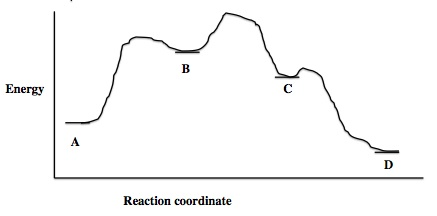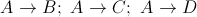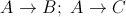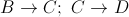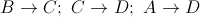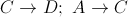Explanation:

An exothermic process, by definition, involves a reaction in which the products are lower in energy than the reactants. The reduction in chemical energy results in a release of heat from the reaction.

In the diagram, the path between points is irrelevant. We are simply looking for any instances in which the product point is below the reactant point. Point C has less energy than point B, and point D has less energy than A, B, or C. Transitions from B to C, C to D, or A to D will all result in a reduction of chemical energy, and a release of heat.

### Example Question #6 : Endothermic And Exothermic Reactions

Electronegativity is an important concept in physical chemistry, and often used to help quantify the dipole moment of polar compounds. Polar compounds are different from those compounds that are purely nonpolar or purely ionic. An example can be seen by contrasting sodium chloride, NaCl, with an organic molecule, R-C-OH. The former is purely ionic, and the latter is polar covalent.

When comparing more than one polar covalent molecule, we use the dipole moment value to help us determine relative strength of polarity. Dipole moment, however, is dependent on the electronegativity of the atoms making up the bond. Electronegativity is a property inherent to the atom in question, whereas dipole moment is a property of the bond between them.

For example, oxygen has an electronegativity of 3.44, and hydrogen of 2.20. In other words, oxygen more strongly attracts electrons when in a bond with hydrogen. This leads to the O-H bond having a dipole moment.

When all the dipole moments of polar bonds in a molecule are summed, the molecular dipole moment results, as per the following equation.

Dipole moment = charge * separation distance

A scientist decides that the polar nature of water means that it will be useful to help him investigate the solution chemistry of a salt. He dissolves the salt in water, and is surprised to find that the beaker becomes very hot to the touch. Which of the following is likely true of this reaction?

Assume the system is defined as the beaker with the solute and solvent.

More energy is released when hydrated ions form than is absorbed when ionic bonds break

Equal energy is released when hydrated ions form than is absorbed when ionic bonds break

Entropy of the system must increase

Less energy is released when hydrated ions form than is absorbed when ionic bonds break

The reaction is strongly endothermic

More energy is released when hydrated ions form than is absorbed when ionic bonds break

Explanation:

Energy is always released when bonds form, and always absorbed when bonds break. In the situation described in the question, heat is released when the salt dissolves because energy is released when the water forms dipole-based bonds with the newly dissolved ions. This energy is larger in magnitude than the energy that is absorbed to break the ionic bonds in the salt, thus, there is a net flow of heat out of the system.

The entropy of the system must not necessarily increase in this case, because the entropy of the universe is increasing owing to the release of heat. This could be enough to offset a local decrease in entropy in the system.

### Example Question #7 : Endothermic And Exothermic Reactions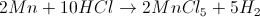5.6g of manganese reacts with 650mL of 6.0M hydrochloric acid to form manganese (V) chloride and hydrogen gas. Along with the products, a large amount of heat is evolved.

This reaction is classified as __________.

Endothermic, with a positive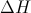Exothermic, with a positiveEndothermic, with a negativeExothermic, with a negativeExothermic, with a negativeExplanation:

Any reaction in which heat is evolved (released) is classified as exothermic. The change in heat content () is negative for this type of reaction.

### Example Question #8 : Endothermic And Exothermic Reactions

A scientist prepares an experiment to demonstrate the second law of thermodynamics for a chemistry class. In order to conduct the experiment, the scientist brings the class outside in January and gathers a cup of water and a portable stove.

The temperature outside is –10 degrees Celsius. The scientist asks the students to consider the following when answering his questions:

Gibbs Free Energy Formula:

ΔG = ΔH – TΔS

Liquid-Solid Water Phase Change Reaction:

H2O(l) ⇌ H2O(s) + X

The scientist prepares two scenarios.

Scenario 1:

The scientist buries the cup of water outside in the snow, returns to the classroom with his class for one hour, and the class then checks on the cup. They find that the water has frozen in the cup.

Scenario 2:

The scientist then places the frozen cup of water on the stove and starts the gas. The class finds that the water melts quickly.

After the water melts, the scientist asks the students to consider two hypothetical scenarios as a thought experiment.

Scenario 3:

Once the liquid water at the end of scenario 2 melts completely, the scientist turns off the gas and monitors what happens to the water. Despite being in the cold air, the water never freezes.

Scenario 4:

The scientist takes the frozen water from the end of scenario 1, puts it on the active stove, and the water remains frozen.

In scenario 2, the reaction that water undergoes would best be characterized as __________.

exothermic in this scenario, and nonspontaneous at current conditions

endothermic in this scenario, and nonspontaneous at standard conditions

exothermic in this scenario, and spontaneous at standard conditions

endothermic in this scenario, and spontaneous at standard conditions

exothermic in this scenario, and nonspontaneous at standard conditions

endothermic in this scenario, and nonspontaneous at standard conditions

Explanation:

The "reaction that water undergoes" is melting. Melting is endothermic, as the reaction written in the pre-question passage illustrates. Standard conditions are defined as 0C and 1 atm of pressure. Water freezes at 0C, and thus the melting reaction is nonspontaneous at these conditions.

### Example Question #9 : Endothermic And Exothermic Reactions

A system experiences the following energetic changes: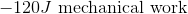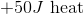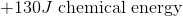Which of the following is true?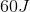of energy is input into the system

The system losesof energy

The system loses heat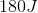of energy is input into the systemof energy is input into the system

Explanation:

Remember that a negative sign denotes an exothermic process (heat is released into the surroundings), while a positive energy change denotes an endothermic process (heat is absorbed).

Using the given values we can calculate the total energy change of the system: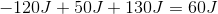Since the overall energy change is positive,of energy is input into the system rather than lost to the surroundings.

### Example Question #10 : Endothermic And Exothermic Reactions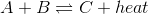Is the reaction above endothermic or exothermic?

Endothermic because an increase in temperture would cause the reaction to shift to the right

Endothermic because an increase in temperture would cause the reaction to shift to the left

Exothermic because an increase in temperture would cause the reaction to shift to the left

Exothermic because an increase in temperture would cause the reaction to shift to the right